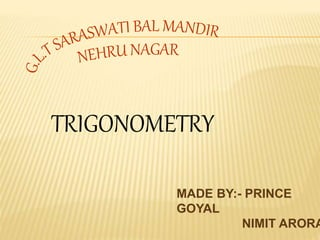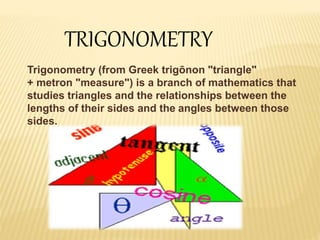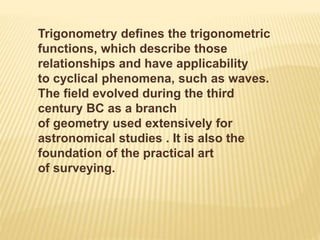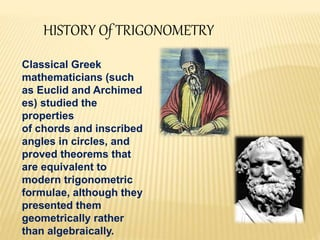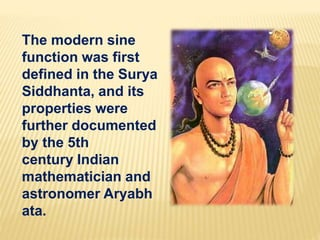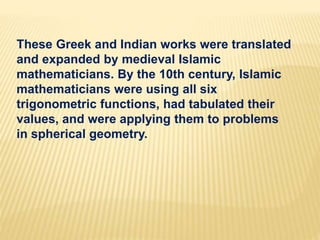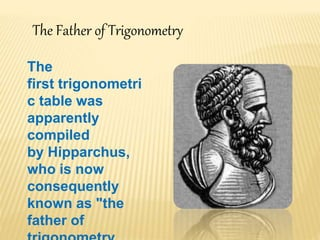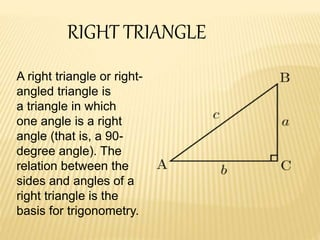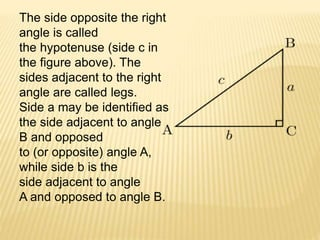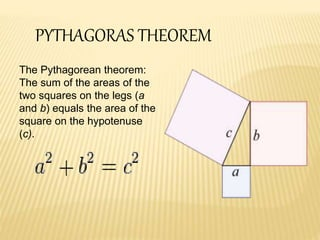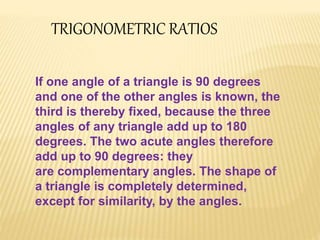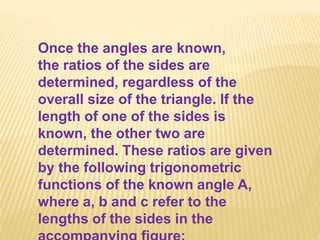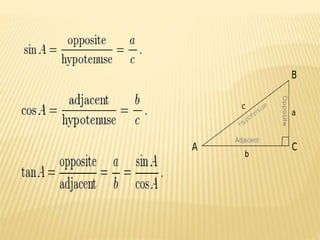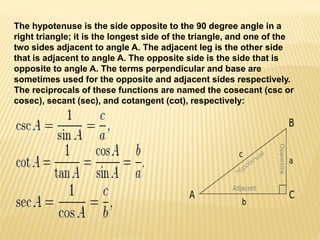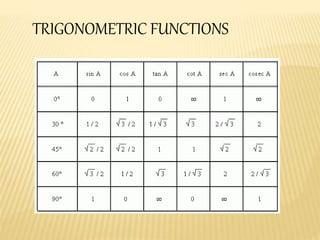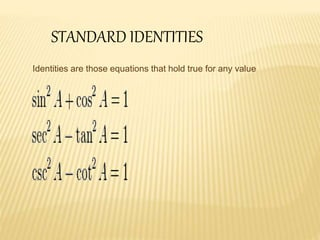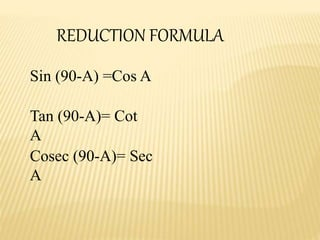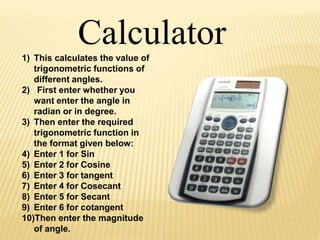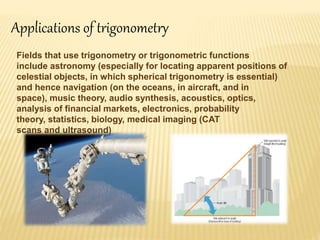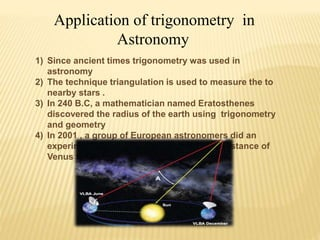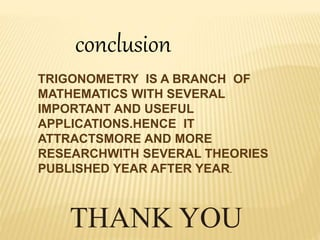1 of 21

### Trigonometry

1. TRIGONOMETRY MADE BY:- PRINCE GOYAL NIMIT ARORA
2. TRIGONOMETRY Trigonometry (from Greek trigōnon "triangle" + metron "measure") is a branch of mathematics that studies triangles and the relationships between the lengths of their sides and the angles between those sides.
3. Trigonometry defines the trigonometric functions, which describe those relationships and have applicability to cyclical phenomena, such as waves. The field evolved during the third century BC as a branch of geometry used extensively for astronomical studies . It is also the foundation of the practical art of surveying.
4. HISTORY Of TRIGONOMETRY Classical Greek mathematicians (such as Euclid and Archimed es) studied the properties of chords and inscribed angles in circles, and proved theorems that are equivalent to modern trigonometric formulae, although they presented them geometrically rather than algebraically.
5. The modern sine function was first defined in the Surya Siddhanta, and its properties were further documented by the 5th century Indian mathematician and astronomer Aryabh ata.
6. These Greek and Indian works were translated and expanded by medieval Islamic mathematicians. By the 10th century, Islamic mathematicians were using all six trigonometric functions, had tabulated their values, and were applying them to problems in spherical geometry.
7. The Father of Trigonometry The first trigonometri c table was apparently compiled by Hipparchus, who is now consequently known as "the father of
8. RIGHT TRIANGLE A right triangle or right- angled triangle is a triangle in which one angle is a right angle (that is, a 90- degree angle). The relation between the sides and angles of a right triangle is the basis for trigonometry.
9. The side opposite the right angle is called the hypotenuse (side c in the figure above). The sides adjacent to the right angle are called legs. Side a may be identified as the side adjacent to angle B and opposed to (or opposite) angle A, while side b is the side adjacent to angle A and opposed to angle B.
10. PYTHAGORAS THEOREM The Pythagorean theorem: The sum of the areas of the two squares on the legs (a and b) equals the area of the square on the hypotenuse (c).
11. If one angle of a triangle is 90 degrees and one of the other angles is known, the third is thereby fixed, because the three angles of any triangle add up to 180 degrees. The two acute angles therefore add up to 90 degrees: they are complementary angles. The shape of a triangle is completely determined, except for similarity, by the angles. TRIGONOMETRIC RATIOS
12. Once the angles are known, the ratios of the sides are determined, regardless of the overall size of the triangle. If the length of one of the sides is known, the other two are determined. These ratios are given by the following trigonometric functions of the known angle A, where a, b and c refer to the lengths of the sides in the
13. The hypotenuse is the side opposite to the 90 degree angle in a right triangle; it is the longest side of the triangle, and one of the two sides adjacent to angle A. The adjacent leg is the other side that is adjacent to angle A. The opposite side is the side that is opposite to angle A. The terms perpendicular and base are sometimes used for the opposite and adjacent sides respectively. The reciprocals of these functions are named the cosecant (csc or cosec), secant (sec), and cotangent (cot), respectively:
14. TRIGONOMETRIC FUNCTIONS
15. STANDARD IDENTITIES Identities are those equations that hold true for any value
16. REDUCTION FORMULA Sin (90-A) =Cos A Tan (90-A)= Cot A Cosec (90-A)= Sec A
17. Calculator1) This calculates the value of trigonometric functions of different angles. 2) First enter whether you want enter the angle in radian or in degree. 3) Then enter the required trigonometric function in the format given below: 4) Enter 1 for Sin 5) Enter 2 for Cosine 6) Enter 3 for tangent 7) Enter 4 for Cosecant 8) Enter 5 for Secant 9) Enter 6 for cotangent 10)Then enter the magnitude of angle.
18. Applications of trigonometry Fields that use trigonometry or trigonometric functions include astronomy (especially for locating apparent positions of celestial objects, in which spherical trigonometry is essential) and hence navigation (on the oceans, in aircraft, and in space), music theory, audio synthesis, acoustics, optics, analysis of financial markets, electronics, probability theory, statistics, biology, medical imaging (CAT scans and ultrasound)
19. Application of trigonometry in Astronomy 1) Since ancient times trigonometry was used in astronomy 2) The technique triangulation is used to measure the to nearby stars . 3) In 240 B.C, a mathematician named Eratosthenes discovered the radius of the earth using trigonometry and geometry 4) In 2001 , a group of European astronomers did an experiment that started in 1997 about the distance of Venus from the sun.
20. conclusion TRIGONOMETRY IS A BRANCH OF MATHEMATICS WITH SEVERAL IMPORTANT AND USEFUL APPLICATIONS.HENCE IT ATTRACTSMORE AND MORE RESEARCHWITH SEVERAL THEORIES PUBLISHED YEAR AFTER YEAR. THANK YOU